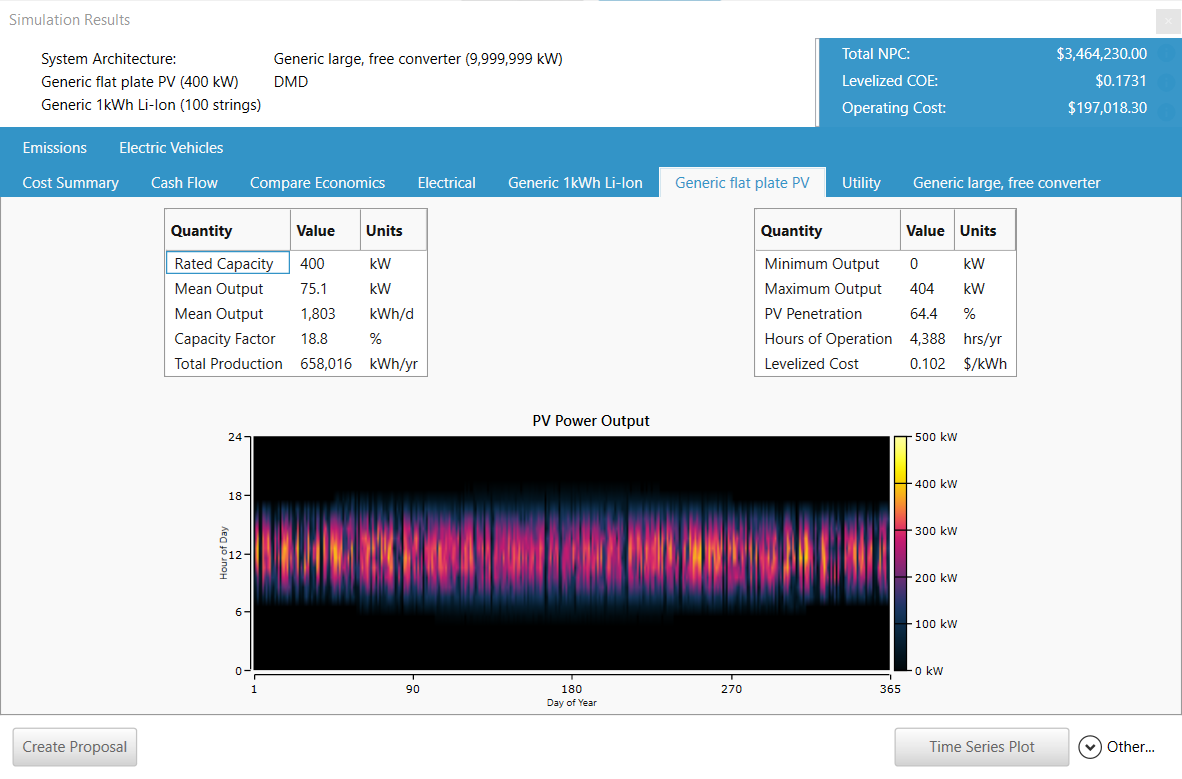HOMER Grid 1.8

 Navigation: Results > Tables Mode > Simulation Results PV OutputsThe PV tab in the Simulation Results window contains the following output variables.

 Variable Description Rated Capacity The rated capacity of the PV array under standard conditions, in kW Mean Output The average power amount of the PV array over the year, in kW and kWh/day Capacity Factor The average power output of the PV array (in kW) divided by its rated power, in % Total Production The total power output of the PV array over the year, in kWh/yr Minimum Output The minimum power output of the PV array over the year, in kW Maximum Output The maximum power output of the PV array over the year, in kW PV Penetration The average power output of the PV array divided by the average primary load, in % Hours of Operation The number of hours of the year during which the PV array output was greater than zero Levelized Cost The levelized cost of energy of the PV array, in \$/kWh

In the bottom half of the page a DMap appears showing the power output of the PV array in each time step of the year.

Below is an example of PV output in the HOMER Grid:The Homer Support site has a searchable knowledgebase and additional support options. HOMER Online contains the latest information on model updates, as well as sample files, resource data, and contact information.UL LLC © 2022